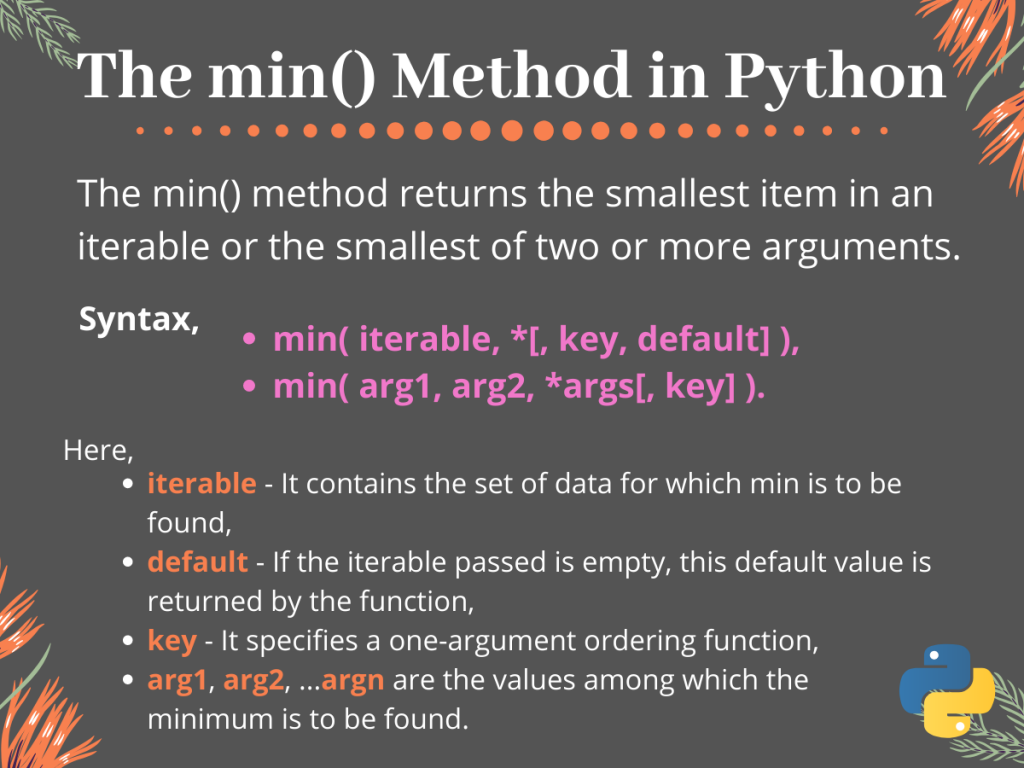# The Python min() Method## Introduction

In this tutorial, we are going to understand the use of the Python min() method.

Basically, the Python `min()` method returns the minimum value among the set of passed values or the elements of the passed iterable.

## Understanding the Python min() Method

The general syntax for using the `min()` method in Python is given below. Using this we can find the minimum value among elements of an iterable( list, tuple, string, etc.).

```min(iterable, *[, key, default])
```

And for finding the minimum value among a set of items, we can directly pass all of them to the `min()` function separated by commas(“, “).

```min(arg1, arg2, *args[, key])
```

Here,

• iterable contains the values for which the smallest has to be found,
• key is a one-line ordering function,
• default is the default value returned by the function if the iterable passed is empty,
• arg1, arg2, … argn are the set of values for which the min() function would return the smallest value.

Now that we understood the syntax for using the `min()` method, let us look at some examples to get a better understanding of it’s working.

## Using the Python min() Method

As mentioned earlier, we can use the Python `min()` function to find the smallest value among a set of values passed as arguments or among elements of the passed iterable.

Now to understand the working we take some examples.

### 1. With Iterable Object

The `min()` function is widely used to find the smallest value present in an iterable like list, tuple, list of lists, list of tuples, etc. In the case of simple lists and tuples, it returns the smallest value present in the iterable.

Look at the example given below.

```# initialisation of list
list1 = [23,45,67,89]

# finding min element
print("Min value is : ", min(list1, default=0))
```

Output:

```Min value is : 23
```

Here, passing the list list1 directly to the `min()` method gives us the minimum of all the elements present in the list i.e., 23. The `default` value is set to 0 so that if the passed iterable was an empty one, the method would have returned this default value(0).

For a list of characters, the `min()` method returns the element with minimum ASCII value.

### 2. With Multiple Arguments

When we pass multiple arguments to the `min()` method, it returns back the smallest of them all.

Note, we can pass multiple values as well as multiple iterables to the `min()` method. For multiple iterables, the method returns the one with the smallest first element (value at 0th index).

The example below explains that easily:

```# initialisation of lists
list1 = [23,45,67]
list2 = [89,65,34]
list3 = [19,90,31]

# finding min element
print("Min among set of values is : ", min(765,876,434))
print("Min list among the given lists is : ", min(list1,list2,list3))
```

Output:

```Min among set of values is :  434
Min list among the given lists is :  [19, 90, 31]
```

For the above example, when we pass multiple values as arguments to the `min()` method, it simply returns us the smallest value(434)

Whereas, for list1, list2 and list3, it returns list3 since it has the minimum 0th index value(19).

### 3. With key function

As we have mentioned earlier, the key function is a one-line ordering function that determines on the basis of which parameter the minimum is to be returned.

Let us take an example to understand this key concept.

```# initialisation of variables
list_of_tuples = [(9, 2, 7), (6, 8, 4), (3, 5, 1)]

list1 = [23,45]
list2 = [89,65,34]
list3 = [19,90,31,67]

def ret_2nd_ele(tuple_1):
return tuple_1

#find Min from a list of tuples with key on the basis of the 2nd element
print("Min in list of tuples : ", min(list_of_tuples, key=ret_2nd_ele))

#find min from a bunch of lists on the basis of their length
print("List with min length : ", min(list1,list2,list3,key=len))
```

Output:

```Min in list of tuples :  (9, 2, 7)
List with min length :  [23, 45]
```
• We at first initialize a list of tuples along with three other integer lists of different lengths,
• Then we define a function `ret_2nd_ele()` that returns the 2nd element or 1st index item of the passed tuple,
• After that, we pass the list_of_tuples to the `min()` method with the `ret_2nd_ele()` function as the key,
• Again we pass the three list list1, list2, and list3 as arguments to the `min()` method with a key set as built-in `len()` method.

In this way, we get the tuple with a minimum 2nd element(1st item) for the list of tuples. And the list with minimum length (using `len()`) among the three lists, i.e. `list1`.

## Conclusion

Always remember that passing an empty iterable with no default value set to the `min()` method, raises a `ValueError`.

So that was it for this tutorial on `min()` method in Python. For any further questions, feel free to use the comments below.